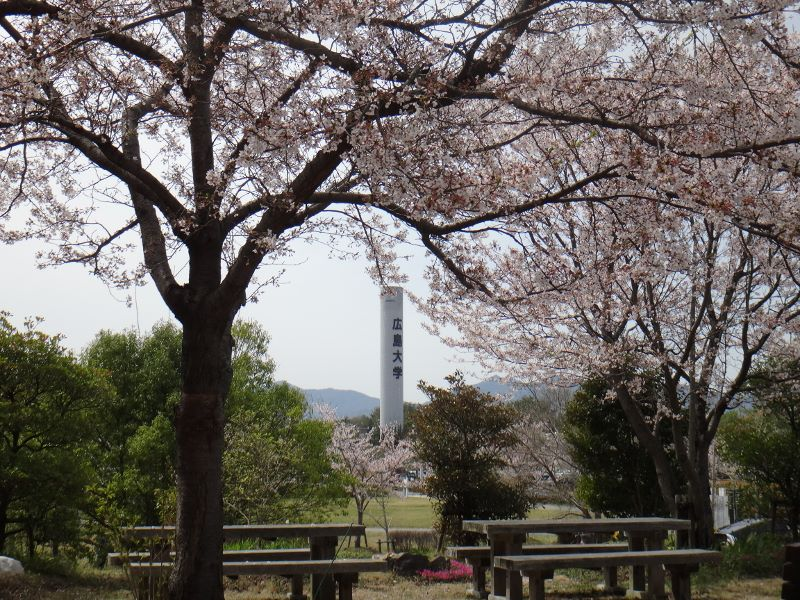# Kentaro Hirata

• Associate Professor
• Department of Mathematics,
Hiroshima University,
Higashi-Hiroshima 739-8526, Japan
• E-mail: hiratake♦hiroshima-u.ac.jp (Replace ♦ by @)

### Research interests

• Potential theory for Laplace's equation in Rn
• Potential analysis for semilinear elliptic equations

### Some recent research papers

• (Joint work with A. Seesanea), The Dirichlet problem for sublinear elliptic equations with source, Bull. Sci. Math. (to appear)
• Boundary growth rates and exceptional sets for superharmonic functions on the real hyperbolic ball, J. Geom. Anal. (to appear)
• Boundary growth rates and the size of singular sets for superharmonic functions satisfying a nonlinear inequality, Arch. Math., vol. 116, pp. 335--344, (2021)
• (Joint work with H. Aikawa and T. Hara), Global integrability of supertemperatures, Math. Z., vol. 296, pp. 1049--1063, (2020)
• Boundary estimates for superharmonic functions and solutions of semilinear elliptic equations with source, Collect. Math., vol. 72, pp. 43--61, (2021)
• A priori growth estimates for nonnegative supertemperatures and solutions of semilinear heat equations in a Lipschitz domain, J. Anal. Math., vol. 138, pp. 441--463, (2019)

Click the list of all papers and the list of talks (Japanese), if you want to see. Also, preprints of the papers can be obtained there.